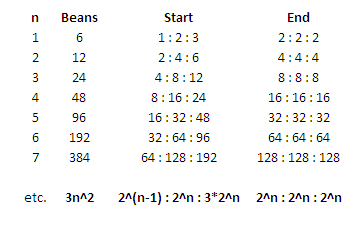BrainDen.com - Brain Teasers
• 0

# jelly beans revisited - achieving equality

## Question

In a previous puzzle @plasmid found that by successively doubling the jelly beans on a plate by transferring from one of the other of three plates, it's possible to empty one of the plates.

Suppose the starting number of jelly beans distributed among three plates is a sufficiently nice multiple of 3, namely b = 3x2n. By making successive doubling moves, as in the first puzzle, is it always possible to end up with an equal number ( 2n ) of jelly beans on the three plates?

## Recommended Posts

• 0

i think so.

for the first number n, n= 1, its fairly trivial, as rocdocmac showed. for larger values, the goal is to get it in a ratio of 1, 2 ,3. to do this, first, let's assume they are in some other configuration, with a<b<c. if a = 1, this is also trivial, we can use the binary approach until a,b c is in the right ratio. if a > 1 we can divide b/a and throw out remainder,  then use the the binary approach before.

for example, lets say  thee are 48 total beans, with 5 on a, 18 on b, and 25 on c. 18/5 = 3, so the 1's digit on b is 1, so transfer from b to a. a = 10 , b = 13, c = 25. now transfer from b to a one more time, a = 20, b = 3, c = 25  C/B, 8, so transfer from a to b, a = 17, b = 6 c = 25, a to b, a = 11, b = 12, c = 25. then form c to b,  a = 11, b = 24, c = 13.  b is now where we want. than transfer from c to a, a = 22, b = 24 c = 2. then form a to c. a = 20 b = 24 c = 4 a  = 16, b = 24 c = 8.

##### Share on other sites

• 0
Spoiler

Kicking off with ... yes, if the distribution ratio on the 3 plates is 1:2:3

Edited by rocdocmac
moved to spolier
##### Share on other sites

• 0
14 hours ago, rocdocmac said:
Hide contents

Kicking off with ... yes, if the distribution ratio on the 3 plates is 1:2:3

Good start. Is it always possible to achieve a distribution ratio of 1:2:3?

##### Share on other sites

• 0

Certainly!

Spoiler##### Share on other sites

• 0

Did OP leave out the part about starting from a random distribution of the (total number of) b = 3x2n beans on the plates? Apologies.

## Join the conversation

You can post now and register later. If you have an account, sign in now to post with your account.×   Pasted as rich text.   Paste as plain text instead

Only 75 emoji are allowed.

×   Your previous content has been restored.   Clear editor

×   You cannot paste images directly. Upload or insert images from URL.